Next: Linear Vector Spaces in Up: Mathematical Background Previous: Unitary Operators

## Commutators in Quantum Mechanics

The commutator, defined in section 3.1.2, is very important in quantum mechanics. Since a definite value of observable A can be assigned to a system only if the system is in an eigenstate of
, then we can simultaneously assign definite values to two observables A and B only if the system is in an eigenstate of bothand
. Suppose the system has a value of Ai for observable A and Bj for observable B. The we require
(64)
If we multiply the first equation byand the second bythen we obtain(65)
and, using the fact that
is an eigenfunction ofand, this becomes
(66)
so that if we subtract the first equation from the second, we obtain(67)

For this to hold for general eigenfunctions, we must have
, or
. That is, for two physical quantities to be simultaneously observable, their operator representations must commute.

Section 8.8 of Merzbacher  contains some useful rules for evaluating commutators. They are summarized below.
(68)
(69)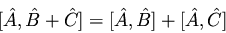(70)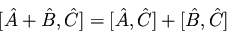(71)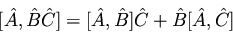(72)(73)
(74)

Ifandare two operators which commute with their commutator, then
(75)
(76)

We also have the identity (useful for coupled-cluster theory)
(77)

Finally, if
then the uncertainties in A and B, defined as
, obey the relation1
(78)

This is the famous Heisenberg uncertainty principle. It is easy to derive the well-known relation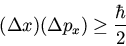(79)

from this generalized rule.Next: Linear Vector Spaces in Up: Mathematical Background Previous: Unitary Operators